# 15.10 - Interval Histograms - Teradata Database

## Teradata Database SQL Request and Transaction Processing

prodname
vrm_release
15.10
category
Programming Reference
User Guide
featnum
B035-1142-151K

Generically defined, a histogram is a count of the number of occurrences, or cardinality, of a particular category of data that fall into defined disjunct value range categories. These categories are typically referred to as bins or buckets.

A synopsis data structure is a data structure that is substantially smaller than the base data it represents. Interval histograms (Kooi, 1980) are a one‑dimensional form of synopsis data structure that provide a statistical and demographic profile of attribute values characterizing the properties of the raw data.

Teradata Database uses equal‑height, high‑biased, and history interval histograms to represent the cardinalities and other statistical values and demographics of columns and indexes for all‑AMPs sampled statistics and for full‑table statistics (see “Interval Histogram Terminology” on page 163 and “Types of Interval Histograms Used By Teradata Database” on page 167 for details). The greater the number of intervals in a histogram, the more accurately it can describe the distribution of data by characterizing a smaller percentage of its composition per each interval. Each interval histogram in the system is composed of a number of intervals (the default is 250 and the maximum is 500) intervals. A 500 interval histogram permits each interval to characterize roughly 0.25% of the data.

Teradata Database determines the number of biased values and equal‑height intervals that can be accommodated for a histogram BLOB with maximum size 64 KB by leaving space for history records. The minimum number of equal‑height intervals for any histogram is 10, and the maximum number is 500. There is no maximum number of biased values in a histogram.

See “Content and Storage of Histograms” on page 171 for more detailed information about the structure of the interval histograms used by Teradata Database.

You can determine the number of intervals used for a histogram by specifying the MAXINTERVALS USING option when you collect statistics.

The number of intervals used to store statistics is a function of the number of distinct values in the column set represented. For example, if there are only 10 unique values in a column or index set, Teradata Database does not store the statistics for that column across 500 intervals, but across 11 (the master record plus the 500 - or 10 - additional intervals containing summary data for the distinct values in the set).

Teradata Database employs the maximum number of intervals for a synopsis data structure only when the number of distinct values in the column or index set for which statistics are being captured equals or exceeds the maximum number of intervals.

The statistical and demographic information maintained in the histograms is used to estimate various attributes of a query, most importantly the cardinalities of various aspects of the relations that are specified in the request.

Conceptually, the cardinality of each interval can be determined from the area under the curve of each interval in the histogram.

The use of interval histograms to make these estimates is a nonparametric statistical method. Nonparametric statistical analyses are used whenever the population parameters of a variable, such as its distribution, are not known.

See Ioannidis (2003) for a brief history of histograms and their use in query optimization for relational database management systems.

Note that Teradata Database also uses derived statistics to estimate cardinalities and selectivities. These statistics are based on the initial values stored in the interval histograms, but are then adjusted for accuracy at each stage of query optimization by incorporating additional information such as CHECK and referential integrity constraints, query predicates, and hash and join indexes. See “Derived Statistics” on page 210 for details).

It should be apparent that the statistics contained in interval histograms are, by their very nature, out of date almost as soon as they have been collected. As a result, they inevitably present an inaccurate summary of the current statistical characteristics of the database.

Ioannidis and Christodoulakis (1993, page 710) write, “Histograms are the most common type of maintained statistics containing the number of tuples in a relation for each of several subsets of values (buckets) in an attribute. Usually, the information contained in a histogram represents an inaccurate picture of the actual contents of the database. This is due to 2 reasons: first, for each subset of values in an attribute, only aggregate information is captured in the histogram; second, as the database is updated, the information becomes obsolete if it is not appropriately updated as well. Hence, the query optimizer uses erroneous data to accomplish its task.”

Pursuing this still further, Antoshenkov (1993, page 538) writes, “Ioannidis and Christodoulakis [(1991)] … demonstrated that the cardinality error of an n-way join grows exponentially with n even if we have good estimates of the number of records delivered by the table scans. It implies that the cost of different execution plans calculated with the industry‑standard cost model … [(Selinger, 1979)] … often has no validity, and thus, the "best" query execution plan is picked quite at random on the scale of real execution costs.”

Both of these quotations are exaggerations, but they do capture the nature of the problems faced by attempting to maintain up‑to‑date statistics for any database management system. The Teradata query optimizer uses derived statistics to reduce the worst‑case error of estimating query cardinalities at the various stages of optimization (see “Derived Statistics” on page 210) and provides several threshold and sampling options for collecting statistics that enable you to avoid recollecting statistics that do not need to be refreshed (see “COLLECT STATISTICS (Optimizer Form)” in SQL Data Definition Language for more information).

The terms used to describe the intervals and histograms used by Teradata Database are defined in the following table.

 Term Definition Bin See “Bucket” in this table. Bucket A synonym for interval in an interval histogram. Cardinality The number of rows per AMP that satisfy a predicate condition. Note that in this context, cardinality generally is not the number of rows in the entire table, but the number of rows that qualify for a predicate filter. See “Using Interval Histograms to Make Initial Cardinality Estimates” on page 198 for a fuller explanation of what cardinality means in this context. Compressed interval histogram A family of histograms that combines high‑biased intervals and equal‑height intervals or high‑biased intervals only, or both, with a master record. When both interval types are present, the high‑biased intervals always precede the equal‑height intervals. A compressed interval histogram can have a maximum of 499 high‑biased intervals. Equal‑depth interval See “Equal‑height interval” in this table. Equal‑depth interval histogram See “Equal‑height interval histogram” in this table. Equal-height interval An interval containing column statistics normalized across the distribution in such a way that the graph of the distribution of the number of rows as a function of interval number is flat. This is achieved by varying the width of each interval so it contains approximately the same number of rows (but with different attribute value ranges) as its neighbors. Equal‑height intervals are also known as equal‑depth intervals. Equal-height interval histogram A family of histograms characterized by approximately equal cardinalities and a variable attribute value range per bucket plus a master record. Also known as equal‑depth interval histograms. More formally, an equal‑height histogram is an array of ordered equal‑height intervals. The definitions for some of the byte fields in equal‑height and high‑biased interval histograms differ as indicated by the following list (see “Content and Storage of Histograms” on page 171 for details). Values is the total number of non‑modal values in the interval. When an equal-height interval represents a single value, the value is saved as a Mode Value. Teradata Database sets the number for Values, which is the total number of non‑model values, to 0 in this case. Mode is the most frequent value in the interval. Modal frequency is the number of rows having the modal value. Maximum value is the maximum value covered by the interval. Rows is the total number of rows for the non‑modal values in the interval. High‑biased interval An interval used to characterize a skewed value set for a column. Any attribute value that is significantly skewed (see the statistic defined under “Loner” in this table) is summarized by a high-biased interval. Each high-biased interval contains statistics for only 1 or 2 attribute row values. High‑biased interval histogram A family of histograms characterized by all loner buckets as seen, for example, with a multimodal or otherwise-skewed attribute value distribution. The definitions for some of the byte fields common to equal-height and high-biased interval histograms differ as indicated by the following table (see “Content and Storage of Histograms” on page 171 for details). Values represents the number of loners in the interval, which is at most 1. Mode is the only loner value in the interval. Modal frequency is the number of rows having the loner value in the interval. Maximum value is the loner value in a high-biased interval. Rows is the number of rows having the loner value in the interval. Histogram A graphic means for representing distributions as a function of the number of elements per an arbitrarily determined interval width. Histograms are often called bar charts. Each bar in a histogram represents the number of rows for the defined interval. Histogram intervals are sometimes referred to as buckets because they contain a number of values that summarize the demographics for the values that fall into the range defined for the interval. In relational query optimization theory, the term is used to describe the rows in a Data Dictionary table or system catalog that store the buckets defined by the particular intervals used to characterize the frequency distribution of the attribute values for a column set. All histograms described in this topic are frequency histograms. Each bucket in a frequency histogram contains some number of tokens representing the number of rows that have the attribute values belonging to its range. Teradata Database stores all interval histograms as BLOBs in the Histogram column of DBC.StatsTbl. History record An interval containing historical information about a histogram including MinValue, MaxValue, HighModeValue, and HighModeFrequency statistics as well as other relevant historical data. When you recollect statistics, Teradata Database saves the summary information from the previous histogram as a history record in the new histogram. Teradata Database determines the number of history records a histogram maintains based on a trend analysis. When history records are no longer needed, the system purges them. Because historical records cannot exist without high‑biased or equal‑height intervals (or both), whose history they record, there are no pure history record histograms. Interval A bounded, non‑overlapping set of attribute value cardinalities. Sometimes called a bin or bucket. Loner A loner is an attribute value whose frequency in the sampled population deviates significantly from a defined criterion; an unusually frequent value indicating significant frequency skew. By definition, only one loner is stored per high-biased interval. A loner is a distinct value that satisfies the following condition.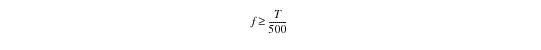where: f defines the frequency of the loner. T defines the cardinality of the relation. Based on the maximum histogram size of 64 KB, Teradata Database determines the number of biased values and equal-height intervals that can be accommodated in a histogram by leaving space for the history records. The system ensures a minimum of 10 equal-height intervals, which can be expanded to the maximum as defined in the COLLECT STATISTICS request, but there is no maximum limit for number of biased values. Master Record A row in DBC.StatsTbl with a StatsId column value of 0 that acts as a statistics identifier within each source column or index statistics set. The Optimizer uses the master record for each column or index set to maintain the cardinality, average row size, dynamic AMP sample estimates, and other object‑level attributes. Whenever statistics are collected or updated for any column or index set, Teradata Database updates the master record of the source to reflect the latest object‑level statistical information. When statistics are refreshed on a column set, the Optimizer resets the UDI counts. Because different statistics can be collected at different times, the current logs of delete and insert counts are retained with the master record before Teradata Database resets them. The UPD counts are retained at the level of individual column statistics. The individual column statistics refer to master record delete and insert counts, column statistics‑level UPD counts, or both, and the latest system UDI counts from DBC.ObjectUsage to determine the overall effective UDI counts for a given statistics. Skew A measure of the asymmetry of the distribution of a set of attribute values or their cardinalities. Skew is the third moment of the probability density function for a population of attribute values. The first 2 moments are the mean and the standard deviation, respectively. With respect to skew in parallel databases, there are several possible types. Attribute value skew refers to skew that is inherent in the data. An example might be a column that can have only 2 values such as TRUE or FALSE. Partition skew refers to skew that results from an uneven distribution of data across the AMPs. The difference is apparent from the context. As used in this book, the term usually refers to the partition skew that occurs when the primary index for a table is defined on a column set that is highly nonunique. Skew is somewhat more likely to be seen with NoPI because of the way the system distributes their rows. Furthermore, the rows inserted by a given request for a FastLoad or Teradata Parallel Data Pump array INSERT operation are always sent to the same AMP (note that you cannot use FastLoad to insert rows into a column‑partitioned table). As more rows are inserted by different requests, the distribution of rows eventually balances among the AMPs, but for small NoPI, where only a few requests are required to load all the rows, the distribution can be skewed. For information about how the Optimizer deals with skew when performing an equality join on skewed tables, see “Strategies for Joining Skewed Tables on an Equality Join Condition” on page 393. Synoptic data structure A data structure that contains summary, or synopsis, metadata.

Depending on the distribution of values, which is also referred to as the degree of skew, in a column or index set, any one of four varieties of histogram types is used to represent its statistics.

Actually, only three interval histogram types are ever used: equal‑height, compressed, and history. Teradata Database never uses pure high‑biased or history interval histograms.

• Equal-height interval histogram
• Piatetsky‑Shapiro and Connell (1984) demonstrated that equal‑height interval histograms have much lower worst‑case and average errors for a wide variety of queries than do equal‑width interval histograms.

The statistics for a column set are expressed as an equal-height interval histogram if none of the frequencies of its values are skewed.

Teradata Database determines the number of biased values and equal‑height intervals that can be accommodated by leaving space for history records. The minimum number of equal‑height intervals for any histogram is 10, and the maximum number is 500.

In an equal-height interval histogram (also referred to as an equal‑depth interval histogram) each interval represents approximately the same number of rows having that range of values, making their ordinate, cardinality, an approximately constant value or height. If a row has a value for a column that is already represented in an interval, then it is counted in that interval, so some intervals may represent more rows than others.

For example, suppose there are approximately one million rows in a table. Then there would be approximately 2,000 rows per interval, assuming a 500 interval histogram.

Suppose that for a given interval, Teradata Database processes the values for 9,900 rows and the next value is present in 300 rows. Those rows would be counted in this interval, and the cardinality for the interval would 10,200. Alternatively, the rows could be counted in the next interval, so this interval would still only represent 9,900 rows.

A COLLECT STATISTICS request divides the rows in ranges of values such that each range has approximately the same number of rows, but it never splits rows with the same value across intervals. To achieve constant interval cardinalities, the interval widths, or value ranges, must vary.

If a histogram contains 500 equal-height intervals, each interval effectively represents a one fifth percentile score (0.20%) for the population of attribute values it represents.

The following graphic illustrates the concept of an equal-height interval histogram. Note that the number of rows per equal‑height interval is only approximately equal in practice, so the graphic is slightly misleading with respect to the precise equality of heights for all equal‑height intervals in the histogram.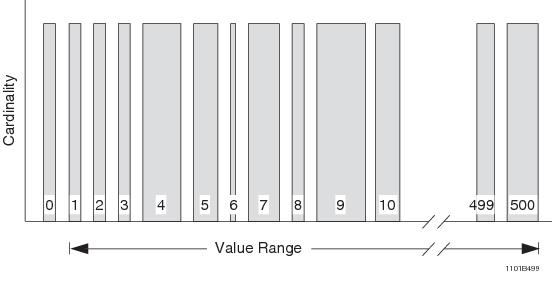• High‑biased interval histogram
• High-biased intervals are used to represent a column or index set only when there is significant skew in the frequency distribution of its values. The Optimizer does not maintain pure high-biased interval histograms. There is no maximum limit for the number of biased values.

Instead, it mixes high-biased intervals with equal-height intervals in a compressed histogram whenever some values for a column or index set are skewed significantly.

In a high-biased interval histogram, each high-biased interval contains only one value.

Note that the number of rows represented by each loner is approximately half the number represented by an equal-height interval in most cases because a loner represents greater than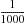, or 0.10% of the rows, while an equal-height histogram represents approximately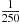, or 0.40% of the rows.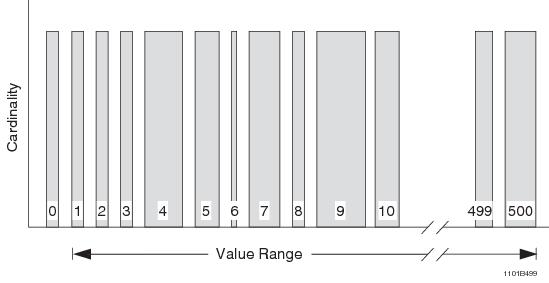• Compressed histogram
• Compressed histograms contain a mix of up to 500 equal- and high‑biased intervals plus a master record, with a maximum of 499 high‑biased intervals plus one equal‑height interval and the master record.

Compressed histograms are an improvement over pure equal‑height histograms because they provide exact statistics for the largest values in the data. This is useful for join estimation, for example, which compares the largest values in relations to be joined.

Beginning at interval 1 and ranging to number_of_intervals - 1, high-biased intervals always precede equal-height intervals in a compressed histogram.

The following graphic illustrates the concept of a compressed histogram with 201 intervals. Note that the number of rows per equal-height interval is only approximately equal in practice, so the graphic is slightly misleading with respect to the precise equality of heights for all equal-height intervals in the compressed histogram.

In this example of a compressed interval histogram, the first three intervals define three loner values and the last several define history intervals.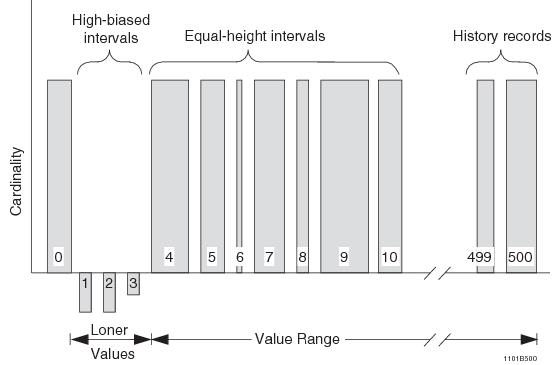• History record
• History records archive a chronicle of the histogram. When you recollect statistics, Teradata Database saves the summary information from the previous histogram as a history interval in the new histogram. The system determines the number of history intervals based on a trend analysis. Teradata Database purges the history intervals that are no longer needed.

A history interval contains MinValue, MaxValue, HighModeValue, HighMode frequency and some additional data.

The number of history intervals maintained for each histogram is based on three criteria.

• The significance of the history interval.
• The determination of number of history records to retain or purge is determined by their significance.

The significance of a history interval depends on the significance of the interval’s participation in the most recent trend analysis. If the significance of a history interval is less than a user‑ or system‑defined threshold, Teradata Database purges it from the histogram.

The significance of a history record is calculated based on the significances of statistical values such as NumRow, UV, AllNull, NumNull, MinVal, and MaxVal from the history interval. The significance of a single statistical value is determined by the following rules.

• If Teradata Database does not use the statistical value in recent trending analyses, its significance is 0.
• If the statistical value does participate in recent trending analyses, its significance is proportional to an absolute difference of the reliabilities with and without the statistical value.
• The minimum number of history records , which Teradata Database sets to 10 by default, indicates the minimum number of history intervals to retain that is determined to be significant. This default is chosen because a minimum of 10 history intervals is required to achieve the necessary reliability factor of a value greater than 0.9.
• The maximum number of history records, which Teradata Database sets to 20 by default, indicates the maximum number of history intervals that are determined to be significant. This default is chosen to be twice the minimum value, which should be sufficient for any trend analysis.
• Teradata Database stores its “interval histograms” as BLOB structures. Because the histogram data is stored as a BLOB, its structure is less accurately defined as a true interval histogram. For example, high‑biased values are not stored in histogram intervals, but as individual data values within the histogram BLOB. The depictions of the histograms described in this chapter frequently use the classic terminology of interval histograms to simplify the explanations, but those representations are not strictly accurate.

The system stores histogram values in a format that is similar to a row structure. All the variable data is stored toward the end of the histogram, and their corresponding offsets are saved in the interval buckets. Data value sizes are constant in the histogram buckets because of the offset usage for variable‑length columns. This enables the histogram buckets to have a fixed size, which makes it possible to randomly position to any bucket.

The length of each variable field is calculated by subtracting its offset from the succeeding variable field offset. The end offset which is saved in the header is used to compute the last variable field length.

A value descriptor which is saved in the histogram header is used to encode and decode the data values of the histogram.

Index and column histograms are stored as BLOBs in the Histogram column of DBC.StatsTbl. Each interval in the histogram has a fixed size to facilitate rapid access.

• Summary statistics are recorded in the histogram header. The header records the following information.
• Collection timestamp
• Statistics version
• Sampling percentage (if the summary statistics were collected by sampling)
• Number of columns
• Number of high‑biased values
• Number of equal‑height intervals
• Number of history intervals
• Number of AMPs
• Number of nulls
• Number of all nulls
• Average AMP NUSI rows per value
• Total number of values and total number of values from partial nulls
• Total number of rows
• High modal frequency
• Offset to the column descriptor
• Offset to the minimum value
• Offset to the high modal value
• Beginning offset to the histogram intervals
• Ending offset for the histogram intervals
• Size of the histogram header
• Use the HELP STATISTICS statement to report the summary statistics for a histogram.

• High-biased intervals store only one count for each biased interval.
• Equal‑height intervals store the following information.
• Maximum value
• Modal value
• Modal value frequency
• Number of non‑modal values
• Lowest frequency among the non‑modal values
• History intervals store the following information.
• Collection timestamp
• Number of high‑biased intervals
• Preceding number of equal‑height intervals
• Usage type
• The possible usage types are as follows.

• Summary
• Detailed
• Sampling percentage (if the statistics were collected by sampling)
• Sampling decision
• Records whether the sampling percentage was determined by a user specification or by a system‑determined sampling percentage.

• Equal‑height interval deviation
• This records the deviation of value frequencies within the equal‑height intervals.

• Number of nulls
• Number of all nulls
• High modal frequency
• Number of distinct values
• Cardinality
• CPU consumed
• I/O consumed
• Use the SHOW STATISTICS statement to report the detailed statistics for a histogram.

Teradata Database uses a value descriptor from the histogram header to encode and decode the data values of the histogram. If the histogram is built on multiple columns, Teradata Database maintains it as an array with each component element describing a single column. The array uses a single element to store single‑column statistics and multiple elements to store multicolumn statistics. If an array element is a variable-length data type, Teradata Database stores a 4-byte offset in the interval for quick access. For fixed length types, the actual data is saved in the intervals. All the variable offsets are saved first followed by the fixed type data.

The histogram header contains all of the summary information and the information to encode and decode the interval buckets. The high‑level structure of a histogram bucket is as follows, with data being stored in the order indicated.

1 Biased values [(value_1), (value_2) …]

2 Biased value frequencies []

3 Equal height values [(Max, Mode) …]

4 Equal height bucket counts [(modal frequency, other Vals, other rows) …]

5 History record values [(Min, Max, HighMode, HighModeFreq) …]

6 History record bucket counts [(OptHistoryIntervalDataType) …]

7 Data values for variable‑length columns.

Teradata Database stores the corresponding offsets to variable‑length columns in the histogram buckets.

There are 3 types of buckets in a Teradata Database interval histogram: biased, equal‑height, and history. There is also the compressed histogram type, which mixes equal‑height and biased intervals.

The offset to each bucket in the histogram is computed from its base offset, which is saved in the histogram header.

• Biased intervals
• Biased intervals are used to represent a column or index set only when there is significant skew in the frequency distribution of its values. The Optimizer does not maintain pure biased interval histograms. Instead, it mixes biased intervals with equal‑height intervals in a compressed histogram whenever some values for a column or index set are skewed significantly.

Teradata Database saves the loner values and their corresponding frequencies for biased intervals in the following general structure.

 Statistic Description Loner value The loner value stored in the interval. Loner value frequency The number of rows that have this loner value.
• Equal‑height intervals
• Piatetsky-Shapiro and Connell (1984) demonstrated that equal-height interval histograms have much lower worst-case and average errors for a wide variety of queries than do equal‑width interval histograms.

The statistics for a column set are expressed as an equal-height interval histogram if none of the frequencies of its values are skewed.

Each equal-height interval contains a modal value for the interval and its frequency, the number of values other than the modal value in the interval, the number of other rows in the interval other than those having the modal frequency, and the lowest frequency among the Other values. Teradata Database uses the lowest frequency to determine the standard deviation in various estimates.

The mode value is saved based on the value descriptor. The frequency and other values/rows are saved in the following general structure.

 Statistic Description Mode frequency The frequency of occurrence of the modal numeric value in the interval. Other values The cardinality of non‑modal values in the interval. Other rows The cardinality of rows other than those having the modal frequency. Lowest frequency The lowest frequency of other (non‑modal) values in the interval.
• Compressed histogram
• Compressed histograms contain a mix of equal-height and biased intervals. Compressed histograms are an improvement over pure equal-height histograms because they provide exact statistics for the largest values in the data. This is useful for join estimation, for example, which compares the largest values in relations to be joined.

• History records
• This interval type records the history of the histogram. When you recollect statistics, Teradata Database saves the summary information from the previous histogram as a history interval in the histogram.

Teradata Database determines how many history intervals to keep based on a linear trend analysis, and purges history records when they are no longer needed.

The general structure of a history record is as follows:

 Statistic Description ANSI timestamp Statistics collection timestamp. Number of biased values Cardinality of the biased values in the histogram. Number of equal‑height intervals Cardinality of the equal‑height intervals in the histogram. Usage type Description of whether the collected statistics are summary or detailed. Sampling percentage If the collected statistics were sampled, states the sampling percentage used. Sampling decision by If sampling was used to collect the statistics, states whether the sampling was system‑determined or user‑determined. Equal‑height interval deviations Deviation of value frequencies within the equal‑height intervals in the histogram. Number of nulls Cardinality of partly null rows in the histogram. Number of all nulls Cardinality of all null rows in the histogram. High modal frequency Frequency of occurrence of the highest modal numeric value in the histogram. Number of values Cardinality of distinct values in the histogram. Number of rows Cardinality of rows in the histogram. CPU cost States how much CPU time was consumed collecting the statistics for the histogram. I/O cost States how much input/output was consumed collecting the statistics for the histogram. None Reserved for future use. None Reserved for future use.

The general structure of an equal‑height interval is as follows.

 Statistic Description Modal frequency Modal value for the interval for numeric data. Other values Number of values other than the modal value. Other rows Number of rows having the modal frequency. Low frequency Lowest frequency among the other values.

The general structure of a history record is as follows.

 Statistic Description Collection time Statistics collection timestamp. Number of biased values Cardinality of the biased history values in the histogram. Number of equal‑height intervals preceding Cardinality of the equal‑height intervals in the histogram. Usage type Description of whether the collected statistics are summary or detailed. Sampling percentage If the collected statistics were sampled, states the sampling percentage used. Sampling decision by If sampling was used to collect the statistics, states whether the sampling percentage was system‑determined or user‑determined. Equal‑height interval deviations Deviation of value frequencies within the equal‑height intervals in the histogram. Number of nulls Cardinality of partly null rows in the histogram. Number of all nulls Cardinality of all null rows in the histogram. High modal frequency The frequency of occurrence of the highest modal numeric value in the histogram. Number of values Cardinality of distinct values in the histogram. Number of rows Cardinality of rows in the histogram. CPU cost States the amount of CPU time that was consumed collecting the statistics for the histogram. I/O cost States the input/output cost that was consumed collecting the statistics for the histogram. None Reserved for future use. None Reserved for future use.

Rows for each histogram are separated into 3 categories.

• Nulls
• Loners
• Non‑loners
• Recall that a histogram can be composed of either of the following types of intervals in addition to a master record.

• 499 high‑biased (loner) intervals and one equal‑height interval
• All equal-height (non‑loner)intervals, referred to as an equal‑height interval histogram.
• A mix of high‑biased, history, and equal-height intervals, referred to as a compressed histogram.
• Teradata Database stores all statistics using the native data type for the column. Because of this, there are no problems with loss of precision, truncation, or with oversized histograms caused by the data types of the values being stored.